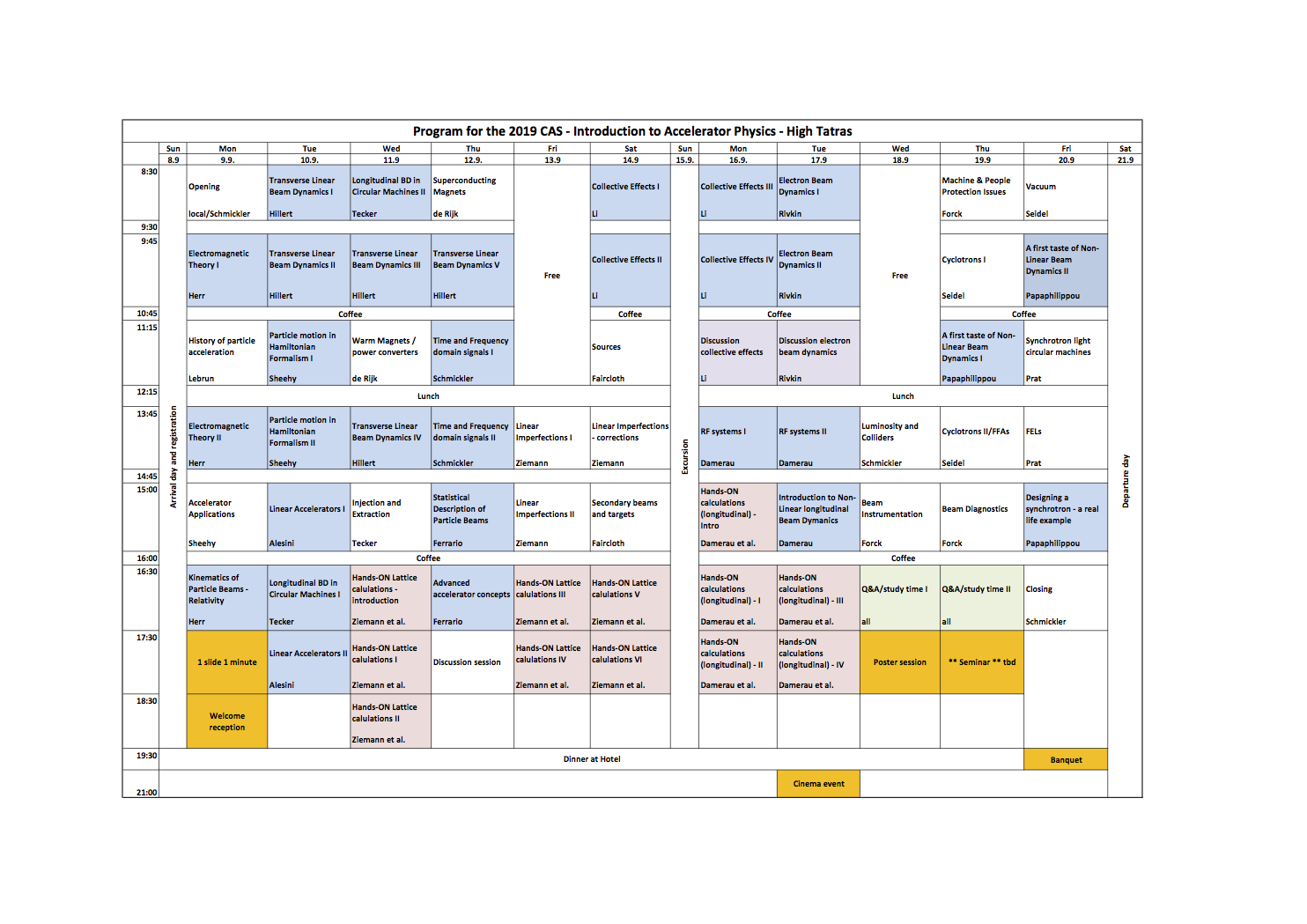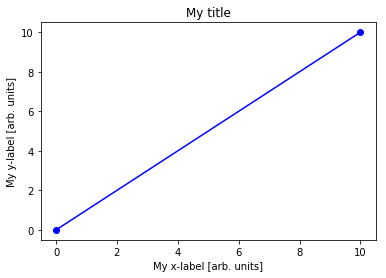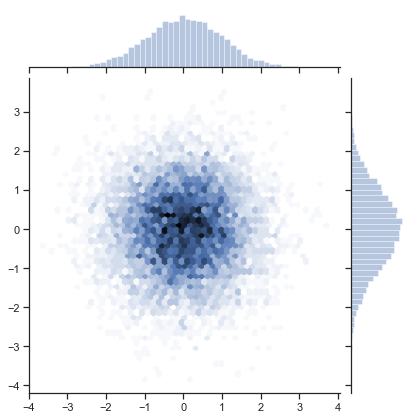# An overview of the Hands-On lattice calculations¶

We have 7 h scheduled this week (https://cas.web.cern.ch/sites/cas.web.cern.ch/files/programmes/vysoke-tatry-2019-programme_5.pdf).

In :
from IPython.display import Image
fig = Image(filename=('/Users/sterbini/CERNBox/2019/CAS/Vysoke_Tatry/Python/Wednesday/programme_5.png'))
fig

Out:The goal is to complement with hand-on numerical exercises the lectures of Transverse Linear Beam Dynamics:

• helping you to visualize the concepts,
• introducing you to the basic numerical approach.

On Wednesday 11.09 we will cover the following topics

• setup of the enviroment and familiarization with python
• first tracking program (1D): definition of the lattice elements, lattice list and beam list
• from a single particle to a beam

On Friday 13.09 we will cover

• tracking of second order moments of the particle ensemble
• periodic solution of the lattice
• matching ("try and error")

On Saturday 14.09 we will generalize the code including

• off momentum (dispersion function, 3x3 matrices)
• 2D motion (horizontal AND vertical plane, 4x4 matrices)
• an example of coupling

We will fine tune the pace depending on the needs.

The format is quite simple: we are going to solve the exercises presented in https://indico.cern.ch/event/808940/contributions/3553546/attachments/1904762/3145489/CAS_Optics_Primer.pdf

You will try first on your own for few minutes and after we will solve and comment together.

The installation procedure for Python3 (it will be used also next week for the longitudinal hands-on) can be found at https://codimd.web.cern.ch/s/HkfQy3YbB

Do not hesitate to ask Werner, Volker, Andrea or Guido if you have questions, doubts or problems!

# An ice-breaker example¶

Just to get familiar with a bit of syntax

In :
# Import the modules
import numpy as np
from matplotlib import pyplot as plt
import seaborn as sns
import sympy as sy

In :
# Matrix definition
Omega=np.array([[0, 1],[-1,0]])
M=np.array([[1, 0],[1,1]])
M

Out:
array([[1, 0],
[1, 1]])
In :
# Sum and multiplication of matrices
Omega - M.T @ Omega @ M
# M.T means the "traspose of M".

Out:
array([[0, 0],
[0, 0]])
In :
aux=np.array([1,2.3,3,])

In :
# Function definition
def Q(f=1):
return np.array([[1, 0],[-1/f,1]])

np.linalg.det(Q(10))

Out:
1.0
In :
np.linalg.det?

Signature: np.linalg.det(a)
Docstring:
Compute the determinant of an array.

Parameters
----------
a : (..., M, M) array_like
Input array to compute determinants for.

Returns
-------
det : (...) array_like
Determinant of a.

--------
slogdet : Another way to represent the determinant, more suitable
for large matrices where underflow/overflow may occur.

Notes
-----

Broadcasting rules apply, see the numpy.linalg documentation for
details.

The determinant is computed via LU factorization using the LAPACK
routine z/dgetrf.

Examples
--------
The determinant of a 2-D array [[a, b], [c, d]] is ad - bc:

>>> a = np.array([[1, 2], [3, 4]])
>>> np.linalg.det(a)
-2.0

Computing determinants for a stack of matrices:

>>> a = np.array([ [[1, 2], [3, 4]], [[1, 2], [2, 1]], [[1, 3], [3, 1]] ])
>>> a.shape
(3, 2, 2)
>>> np.linalg.det(a)
array([-2., -3., -8.])
File:      /anaconda3/lib/python3.7/site-packages/numpy/linalg/linalg.py
Type:      function

In :
# check a simple plot
plt.plot([0,10],[0,10],'ob-')
plt.xlabel('My x-label [arb. units]')
plt.ylabel('My y-label [arb. units]')
plt.title('My title')

Out:
Text(0.5, 1.0, 'My title')In :
# an sns plot
sns.set(style="ticks")
rs = np.random.RandomState(10)
x = rs.normal(size=10000)
y = rs.normal(size=10000)
sns.jointplot(x, y, kind="hex");# Linear Optics and matrices¶

In :
# from Wolfgang's pag.26
fig = Image(filename=('/Users/sterbini/CERNBox/2019/CAS/Vysoke_Tatry/Python/Wednesday/pag26.png'))
fig

Out: